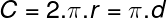# Circle circumference and area calculator

## Circle circumference and area calculations

The circle calculator is designed for calculations of radius, diameter, circumference or area. For successful calculation, you need to know and enter into the calculator at least one of them. Then press send or enter to run calculations.

## Circle parameters sketch:﻿
Unit system:

## Circle calculator variables and constants explanation:

r – radius
C – circumference
d – diameter
A – area
π– 3,1415926535

## Formula for circle circumference calculation:## Formula for circle area calculation:## Circle calculator use:

Circle calculation is used in many industries and in a huge number of calculations. This is at least some of them:

• perimeter of a tree trunk, limb, twig, stem, pipe, tube, hose, duct, wire, rod, cord, thread, rope, tunnel, pillar
• cross-sectional area of a tree trunk, limb, twig, stem, pipe, tube, hose, duct, wire, rod, cord, thread, rope, tunnel, pillar
• partial calculations of geometric shapes like cilinder, cone, sphere, barrel, torus and other rotational shapes
• school calculations